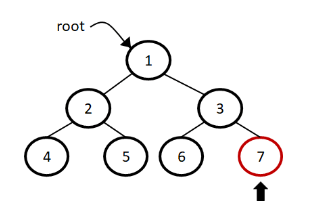# Deepest node in a binary tree

Abhishek Kumar Category: Data StructuresTags: datastructures tree binary-tree

The rightmost node among the leaf nodes is known as the deepest node in a binary tree.
Find the deepest node in a binary tree solution with examples and algorithm.### Deepest node in a tree

The rightmost node among the leaf nodes is known as the deepest node in tree.To find the deepest node in a binary tree we can traverse through all the the nodes in a tree and return the rightmost node among the leaf nodes.

##### Example -
```Input:
1
/   \
2     3
/ \   / \
4   5 6   7
/
8
\
9

Output: 9```

### Solution

For the solution, we have to traverse each node and this can be achieved by using level order traversal and at the end return the deepest node.

###### Algorithm
Deepest_Node(root)
``````1.  if root is null then return null
2.  node = null
3.  queue.enqueue(root)
4.  while queue is not empty
5.      queue.dequeue()
6.      if node.left is not null
7.          queue.enqueue
8.      if node.right is not null
9.          queue.enqueue
10. return node.data``````
``````import queue

class Node:
def __init__(self, data):
self.left = None
self.data = data
self.right = None

def deepest_node(root):
if root is None:
return None

node = None
q = queue.Queue()
q.put(root)

while not q.empty():
node = q.get()
if node.left:
q.put(node.left)
if node.right:
q.put(node.right)
return node.data

root = Node(1)
root.left = Node(2)
root.right = Node(3)
root.left.left = Node(4)
root.left.right = Node(5)
root.right.left = Node(6)
root.right.right = Node(7)
print(deepest_node(root))``````
``````class Node {
constructor(data) {
this.left = null;
this.data = data;
this.right = null;
}
}

function deepestNode(root) {
if (!root) return null;

let node = null;
q = [];
q.push(root);

while (q.length > 0) {
node = q.shift();
if (node.left) q.push(node.left);
if (node.right) q.push(node.right);
}
return node.data;
}

const root = new Node(1);
root.left = new Node(2);
root.right = new Node(3);
root.left.left = new Node(4);
root.left.right = new Node(5);
root.right.left = new Node(6);
root.right.right = new Node(7);
console.log(deepestNode(root));``````

#### Output

Output

`7`

Time complexity: The time complexity of this solution is O(n) because we are just traversing each element of node one by one.

AUTHOR### Abhishek Kumar

Software engineer | Blogger | Keen learner
Python | Django | JavaScript | React | Next.js | Express.js | C
Passionate about learning and experimenting with new things and open to more opportunities and collaborations.